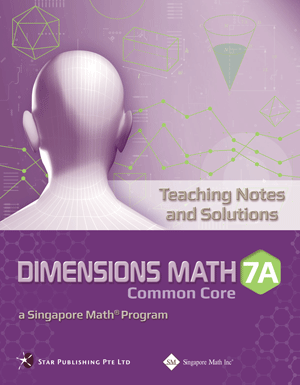# Singapore Math Dimensions Math Teaching Notes and Textbook Solutions 7A

Dimensions Math® Textbook 7A

Chapter 1: Factors and Multiples

1.1 Factors and Multiples

A. Factors
B. Multiples

1.2 Prime Factorization and Exponential Notation

A. Prime Factorization
B. Exponential Notation

1.3 Greatest Common Factor (GCF)
1.4 Least Common Multiple (LCM)
1.5 Square Roots and Cube Roots

A. Square Roots
B. Cube Roots

In A Nutshell
Extend Your Learning Curve
Write In Your Journal

Chapter 2: Real Numbers

2.1 Idea of Negative Numbers and the Number Line

A. Negative Numbers
B. The Number Line
C. Absolute Value

2.2 Addition and Additive Inverse

A. Addition of Integers
B. Additive Inverse

2.3 Subtraction and Absolute Value of the Difference

A. Subtraction of Integers
B. Absolute Value of the Difference

2.4 Multiplication, Division, and Combined Operations of Integers

A. Multiplication of Integers
B. Division of Integers
C. Combined Operations of Integers

2.5 Rational Numbers

A. Definition
B. Addition and Subtraction of Rational Numbers
C. Multiplication and Division of Rational Numbers

2.6 Real Numbers and Use of Calculators

A. Real Numbers
B. Use of Calculators

2.7 Rounding Numbers to Decimal Places

A. Idea of Rounding
B. Rounding Numbers to Decimal Places

In A Nutshell
Extend Your Learning Curve
Write In Your Journal

Chapter 3: Introduction to Algebra

3.1 The Use of Letters in Algebra

A. The Use of Letters
B. Basic Notations in Algebra
C. Exponential Notation

3.2 Evaluation of Algebraic Expressions and Formulas

A. Evaluation of Algebraic Expressions
B. Formulas

3.3 Writing Algebraic Expressions to Represent Real-world Situations

In A Nutshell
Extend Your Learning Curve
Write In Your Journal

Chapter 4: Algebraic Manipulation

4.1 Like Terms and Unlike Terms
4.2 Distributive Law, Addition and Subtraction of Linear Algebraic Expressions

A. Use of Parentheses
B. Addition and Subtraction of Linear Algebraic Expressions

4.3 Simplification of Linear Algebraic Expressions
4.4 Factorization by Extracting Common Factors
4.5 Factorization by Grouping Terms

In A Nutshell
Extend Your Learning Curve
Write In Your Journal

Chapter 5: Simple Equations in One Variable

5.1 Simple Linear Equations in One Variable
5.2 Equations Involving Parentheses
5.3 Simple Fractional Equations
5.4 Forming Linear Equations to Solve Problems

In A Nutshell
Extend Your Learning Curve
Write In Your Journal

Chapter 6: Ratio, Rate, and Speed

6.1 Ratios Involving Rational Numbers

A. Meaning of Ratio
B. Simplification of Ratios
C. Ratio of Three Quantities
D. Problems Involving Ratios

6.2 Average Rate
6.3 Speed

A. Uniform Speed and Average Speed
B. Conversion of Units

In A Nutshell
Extend Your Learning Curve

Chapter 7: Percentage

7.1 Meaning of Percentage

A. Introduction
B. Expressing One Quantity as a Percentage of Another

7.2 Reverse Percentages
7.3 Percentage Increase and Decrease

A. Percentage Increase
B. Percentage Decrease

7.4 Discount and Sales Tax

A. Discount
B. Sales Tax

In A Nutshell
Extend Your Learning Curve
Write In Your Journal

Chapter 8: Angles, Triangles, and Quadrilaterals

8.1 Points, Lines, and Planes
8.2 Angles

A. Angles and Measurements
B. Types of Angles
C. Complementary, Supplementary, and Adjacent Angles
D. Properties of Angles

8.3 Perpendicular Bisectors and Angle Bisectors

A. Use of Compasses
B. Perpendicular Bisectors
C. Angle Bisectors

8.4 Triangles

A. Classification of Triangles
B. Construction of Triangles

8.5 Quadrilaterals

A. Types of Quadrilaterals
B. Properties of Special Quadrilaterals
C. Construction of Quadrilaterals

In A Nutshell
Extend Your Learning Curve
Write In Your Journal

Answers

Note: The answer key in the first printing of Textbook 7A has some errors in it. Please download revised key from here. Please note that not all the answers are incorrect in the first printing answer key, and incorrect answers are highlighted in this corrected answer key. We apologize for the inconvenience caused.

Click here to view Dimensions Math® Teaching Notes and Solutions 7A samples.

Dimensions Math® G6-8 Textbooks are designed for middle school students. Developed in collaboration between Star Publishing and Singapore Math Inc®, this series follows the Singapore Mathematics Framework and covers the topics in the Common Core State Standards.

The emphasis of this series is on empowering the students to learn mathematics effectively and independently. Depending on the topics covered, different approaches are adopted for the presentation of concepts to facilitate easy understanding by students to internalize concepts and instill in them an interest to explore the topics further.

Each book includes appropriate examples, class activities and diagrams to understand the concepts and apply them. IT skills and values are incorporated as appropriate.

Some features of this series include the following:

1. Class activities which allow the student to learn mathematics through discovery. Some of the class activities make use of Geometer’s Sketchpad (available at keypress.com).
2. Worked examples are followed by a similar question (Try It!) so that students can check if they have understood the concepts presented earlier.
3. An exercise for each lesson including questions in the following sequence:
• Basic Practice (simple questions involving a direct application of the concepts)
• Further Practice (more challenging questions on direct application)
• Maths@Work (questions that apply mathematical concepts to real-life situations)
• Brainworks (questions involving higher order thinking or an open-ended approach to problems)
4. Each chapter is followed by a review exercise; the Extend Your Learning Curve activity encourages students to explore mathematical concepts further or apply mathematics in real-life situations, with some questions requiring sentence or paragraph answers to encourage students to reflect on their learning experiences.
5. The right margins contain remarks on important information, concepts or definitions covered earlier related to the current material to help students recall them, discussion questions, interesting puzzles or facts that are related to mathematics, and some website references.

The answer key at the back of the book provides answers to the Try It! and the problems in the exercises for the Basic PracticeFurther Practice, and Maths@Work questions. It does not include answers to the class activities or the Extend Your Learning Curve activities.